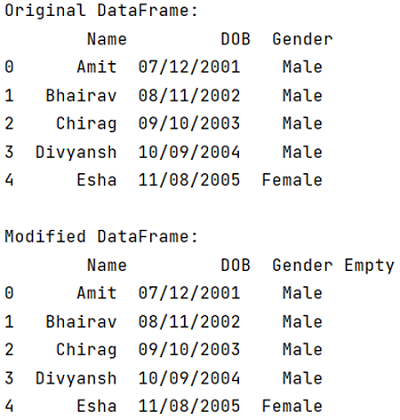# How to Add an Empty Column to a DataFrame?

Add Empty Column to DataFrame: In this tutorial, we will learn how can you add an empty column to a Pandas DataFrame? By Pranit Sharma Last updated : April 19, 2023

## Overview

Columns are the different fields that contain their particular values when we create a DataFrame. We can perform certain operations on both rows & column values. Adding an empty column to the DataFrame is possible and easy as well. Let us understand, how we can add an empty DataFrame to the DataFrame?

## Add an Empty Column to a Pandas DataFrame

To add an empty column in a Pandas DataFrame, simply pass a new column name as an index with a value (if you want) or without a value ("") to the existing DataFrame.

### Syntax

The following code snippet can be used to add an empty column to DataFrame:

```df['col_name'] = "col_value"
#or
df['col_name'] = ""
```

Let us understand with the help of an example.

## Python Program to Add an Empty Column in a DataFrame

```# Importing pandas package
import pandas as pd

# Creating a Dictionary
dict = {'Name':['Amit','Bhairav','Chirag','Divyansh','Esha'],
'DOB':['07/12/2001','08/11/2002','09/10/2003','10/09/2004','11/08/2005'],
'Gender':['Male','Male','Male','Male','Female']}

# Creating a DataFrame
df = pd.DataFrame(dict)

# Display original DataFrame
print("Original DataFrame:\n",df,"\n")

# Adding a new column named Empty and
# passing an empty string as its value
df['Empty'] = " "

# Display modified DataFrame
print("Modified DataFrame:\n",df)
```

### Output